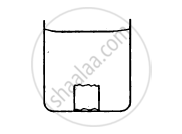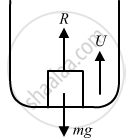Advertisement Remove all ads

# A Metal Piece of Mass 160 G Lies in Equilibrium Inside a Glass of Water. the Piece Touches the Bottom of the Glass at a Small Number of Points. - Physics

Sum

A metal piece of mass 160 g lies in equilibrium inside a glass of water. The piece touches the bottom of the glass at a small number of points. If the density of the metal is 8000 kg/m3, find the normal force exerted by the bottom of the glass on the metal piece.Advertisement Remove all ads

#### Solution

Given:
Mass of the metal piece, m =160 gm = 160 × 10−3 kg
Density of the metal piece, ρm = 8000 kg/m3
Density of the water, ρw = 1000 kg/m3
Let R be the normal reaction and U be the upward thrust.From the diagram, we have:
mg = U + R

⇒ R = mg −Vρwg            [U = Vρwg]

$\Rightarrow \text{R = mg }- \frac{\text{m}}{\rho_\text{m}} \times \rho_\text{w} \times \text{g}$

$= 160 \times {10}^{- 3} \times \left( 10 - \frac{{10}^3 \times 10}{8000} \right)$

$= 160 \times {10}^{- 3} \times 10\left( 1 - \frac{1}{8} \right)$

$= 1 . 4 \text{N }$

Is there an error in this question or solution?
Advertisement Remove all ads

#### APPEARS IN

HC Verma Class 11, 12 Concepts of Physics 1
Chapter 13 Fluid Mechanics
Q 11 | Page 274
Advertisement Remove all ads

#### Video TutorialsVIEW ALL 

Advertisement Remove all ads
Share
Notifications

View all notifications

Forgot password?